# Problems on Quant and Rate Change : CAT Quantitative Aptitude

The topic of ‘Rate of Change’ requires a basic understanding of initial calculus and it has been seen that CAT does throw a problem once in a while on this topic.

The topic of ‘Rate of Change’ requires a basic understanding of initial calculus and it has been seen that CAT does throw a problem once in a while on this topic Let’s see the solved examples to understand the basic nature of problems falling under this topic.

Example:

The radius of a circle increases at a rate of 0.4 cm/s. Then, the rate of increase of the area when the radius is 2 cm is: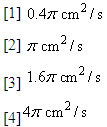Solution:

Let the area be A cm2 and the radius r cm.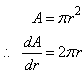That is the area is increasing  times as fast as the radius.

When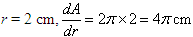So the area is increasing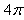times as fast as the radius. But the radius is increasing at 0.4 cm/s.

therefore The area is increasing at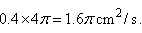Example:

Water is flowing of a conical funnel having a semi-vertical angle of 45º. When the depth of water is 4 cm, the water level is falling by 0.2 cm/s. The volume rate of flow of the water will equal: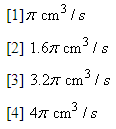Solution:
Let the volume of water be V cm3 when the depth of water is h cm and the radius r cm.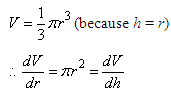(h always equals r because the semi-vertical angle is 45º).

Thus the volume of water is decreasing  times faster than the radius.

When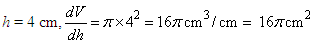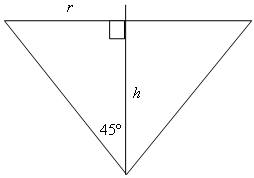Thus V is decreasing 16∏times faster than the height when h = 4 cm. But the height is decreasing by 0.2 cm/s.

therefore The flow rate of water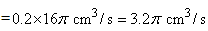Therefore, the correct choice is option .

Two lines represented by the equations: 3x + 2y = 7  and 12y = 17 - 18 x  are drawn on a Cartesian plane. These lines, then, will:

 have a point in common

 be perpendicular to each other

 be parallel to each other

 be collinear

Solution: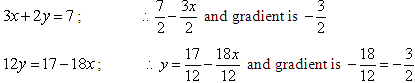Thus the lines are parallel because the gradient of each line is the same. Therefore, the correct choice is option .

Example:

If a line is such that it passes through the points A(2, 5) and B(3, 10), then the slope and y-intercept of this line will, respectively, be:

 -5 and 5

  5 and -1

  -5 and -10

  5 and -5

Solution:

The point (2, 5) lies on the line     y= mx=c

therefore   5 = 2m + c          …(i)

The point (3, 10) lies on the line     y= mx=c

therefore 10 = 3m+c                …(ii)

Subtracting (i) from (ii), m = 5

Substituting m = 5 in (i), 5 = 2x5x+c

c= -5

Therefore The equation of the line is y = 5x-5

Therefore, the correct option is .